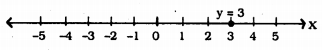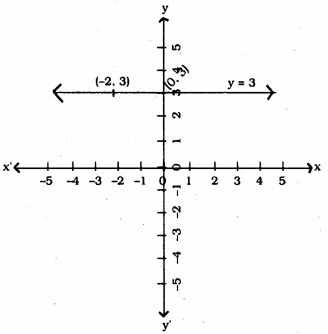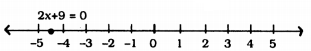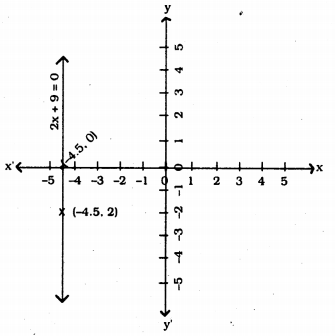# KSEEB Solutions for Class 9 Maths Chapter 10 Linear Equations in Two Variables Ex 10.4

In this chapter, we provide KSEEB SSLC Class 9 Maths Chapter 10 Linear Equations in Two Variables Ex 10.4 for English medium students, Which will very helpful for every student in their exams. Students can download the latest KSEEB SSLC Class 9 Maths Chapter 10 Linear Equations in Two Variables Ex 10.4 pdf, free KSEEB SSLC Class 9 Maths Chapter 10 Linear Equations in Two Variables Ex 10.4 pdf download. Now you will get step by step solution to each question.

## Karnataka Board Class 9 Maths Chapter 10 Linear Equations in Two Variables Ex 10.4

Question 1.
Give the geometric representations of y = 3 as an equation.
(i) in one variable
(ii) in two variables.
Solution:
x + 3 = 0
i) In one variable, y = 3.ii) In two variables, y = 3 straightline passes through (0, 3) parallel to x-axis. For any value of x, value of y is 3.Question 2.
Give the geometric representations of 2x + 9 = 0 as an equation.
(i) in one variable
(ii) in two variables.
Solution:
2x + 9 = 0, this equation in
i) One variable 2x + 9 =0
2x = -9
x = −92 = -4.5ii) 2x + 9 = 0 in two variables, 2x + 9 = 0. This equation passes through (-4.5, 0) and this is parallel ot y-axis. Coordinate of x = – 4.5All Chapter KSEEB Solutions For Class 9 Maths

—————————————————————————–

All Subject KSEEB Solutions For Class 9

*************************************************

I think you got complete solutions for this chapter. If You have any queries regarding this chapter, please comment on the below section our subject teacher will answer you. We tried our best to give complete solutions so you got good marks in your exam.

If these solutions have helped you, you can also share kseebsolutionsfor.com to your friends.

Best of Luck!!